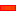﻿ Kinematics | Add Measurement FB

# Kinematics | Add Measurement FB

### Why use a Measurement Function-Block?

Measurement FB:

 • A measurement that is a 'driven dimension' that continually updates as you cycle the mechanism.

You can measure the:

 • Length of a Line
 • Distance between: two Points, a Line* and a Point, two parallel Lines*
 • Angle between: two Points, two Lines*, three Points.

* A Line, can also be a CAD-Line, and the X-axis or Y-axis of a Part.

Measurement FB: Output-Connectors:

 • Two output-connectors when you measure between two Points - the top connector provides the linear motion-values, and the bottom connector provides the angular motion-values.
 • One output-connector for all other measurements.
 • Output-connectors give all motion-derivatives.Kinematics FB toolbar > Measurement FB [right of Graphic-Area >>]

 1 Click Kinematics FB Toolbar > Measurement FB
 2 Click the sketch-element, or sketch-elements, between which you want to measure.
 3 Click the graphic-area to complete the command.

The Measurement FB and the measurement dimension show in the graphic-area.

Use:

Connect a wire from the output-connector of a Measurement FB to:

 1 Plot the motion-values with a Graph FB
 2 Motion-Dimension FB or Motion-Path FB - the Motion-Part or Motion-Point uses the Measurement as its motion.
 3 Motion FB, to become the independent variable [X-axis] for the Motion, and the output will be the corresponding Y-axis of the Motion, as it appears in MotionDesigner..

Note: Options 2 & 3: a message in the Feedback-Area: '1 Mechanism dependencies detected'. This means, that the motion of a motion-axis is a function of a different-motion-axis.

Connecting FBs

## Measurement FB - more details

#### How to Add a Measurement FBMeasrement FB between two Points

 STEP 2: Click sketch-elements in the graphic-area
 STEP 3: Click in the graphic-area to place the Measurement FB.

The Measurement FBis near to the dimension. You can drag the FB to a more convenient location.

In this example: The dimensionis between two PointsThe Measurement FB has two output-connectors when it measures between two Points

The Measurement FB has one output-connector when you measure between other sketch-elements.

See details below for motion-values available from the output-connectors.

####Linear Distance

 • Distance between Two Points
 • Perpendicular Distance between a Point and a Line

Screen-Shot

Sketch-Elements

Measurement DimensionCircle or Arc.

Length: The length of the Line!Two Parallel Lines.

NOT RECOMMENDED

NOT RECOMMENDED...

It is often difficult to measure the distance between two parallel lines.

The Measurement FB will often return the angle between the lines as 0º, 360º or 180º.

Measure from one of the Lines and a Point at the end of one of the Lines.

See Also: Angle Measurement between Two Lines, and Distance between Line and Point.Two Points

Distance between two Points.

The Measurement FB has two output-connectors:

 • Top output-connector: gives the gap, the rate-of-change of the dimension and the second rate-of-change of the dimension
 • Bottom output-connector: gives the angle, angular velocity and angular acceleration between the Points.Point and a Line

Perpendicular Distance between a Line and a Point.

Select the Point, then the Line.

You many need to add a Line to a Part in the Part-Editor.

To measure a 'Horizontal Distance' or a 'Vertical Distance' you can select the X-axis or Y-axis as a Line.

####Angle between Three Points

My work-around when two Points are at one Point, but I want to add a measurement with the Point at the apex of an angle.

 1 Edit the Part and add a 'Free' Line so that its start-Point is near to the coincident Points.
 2 Add the Measurement: Three Points with the Points at each end of the Line, plus another Point.

When you do this, the angle is not yet giving the angle you actually want.

 3 Edit the Part to make one of the Points at the end of the Line to be coincident with the Point you want to be the apex of the angle.

Now, the angle is the angle you want.

Measurement: Angle between Three Points

In the graphic-area:

 1 Click a Point '1'.

Point '1' is the 'apex' of the angle.

 2 Click Point '2'
 3 Click Point '3'.

The Measurement FB displays the counter-clockwise angle from Point '2' to Point '3'.

Screen-Shot

Sketch-Elements

Measurement DimensionThree Points

 1 Click 'Apex' Point2 Click Point3 Click PointInternal Angle

Angle is fromtoin a Counter-Clockwise direction.Three Points

 1 Click 'Apex' Point2 Click Point3 Click PointExternal Angle

Angle is fromtoin a Counter-Clockwise direction.

####Angle between Two LinesAdd Measurement FB : two Lines Eight possible angles

After you select the two Lines, and before you click in the graphic-area to place the Measurement FB and angle dimension, you can display one of eight possible angles.

Move your mouse-pointer around the apex of the two lines:

Counter-clockwise : dimension < 180° , namely, the Acute, Obtuse, Supplementary and Vertical angles,

Clockwise : dimension > 180° : namely, the 'reflex angles' to those < 180°.

Tutorial and Reference Help Files for MechDesigner and MotionDesigner 13.2 + © Machine, Mechanism, Motion and Cam Design Software by PSMotion Ltd